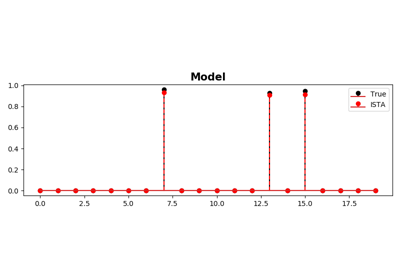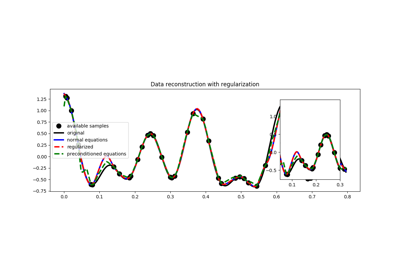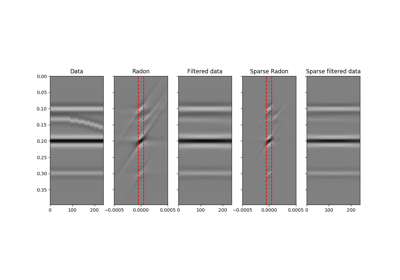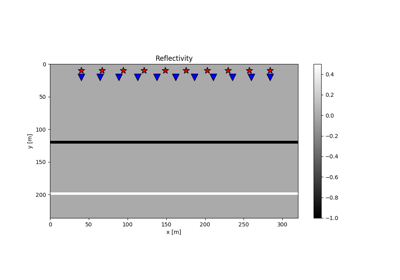# pylops.optimization.sparsity.FISTA¶

pylops.optimization.sparsity.FISTA(Op, data, niter, eps=0.1, alpha=None, eigsiter=None, eigstol=0, tol=1e-10, returninfo=False, show=False)[source]

Fast Iterative Soft Thresholding Algorithm (FISTA).

Solve an optimization problem with $$L1$$ regularization function given the operator Op and data y. The operator can be real or complex, and should ideally be either square $$N=M$$ or underdetermined $$N<M$$.

Parameters: Op : pylops.LinearOperator Operator to invert data : numpy.ndarray Data niter : int Number of iterations eps : float, optional Sparsity damping alpha : float, optional Step size ($$\alpha \le 1/\lambda_{max}(\mathbf{Op}^H\mathbf{Op})$$ guarantees convergence. If None, estimated to satisfy the condition, otherwise the condition will not be checked) eigsiter : int, optional Number of iterations for eigenvalue estimation if alpha=None eigstol : float, optional Tolerance for eigenvalue estimation if alpha=None tol : float, optional Tolerance. Stop iterations if difference between inverted model at subsequent iterations is smaller than tol returninfo : bool, optional Return info of FISTA solver show : bool, optional Display iterations log xinv : numpy.ndarray Inverted model niter : int Number of effective iterations cost : numpy.ndarray, optional History of cost function

ISTA
Iterative Soft Thresholding Algorithm (FISTA).

Notes

Solves the following optimization problem for the operator $$\mathbf{Op}$$ and the data $$\mathbf{d}$$:

$J = ||\mathbf{d} - \mathbf{Op} \mathbf{x}||_2^2 + \epsilon ||\mathbf{x}||_1$

using the Fast Iterative Soft Thresholding Algorithm (FISTA) . This is a modified version of ISTA solver with improved convergence properties and limitied additional computational cost.

  Beck, A., and Teboulle, M., “A Fast Iterative Shrinkage-Thresholding Algorithm for Linear Inverse Problems”, SIAM Journal on Imaging Sciences, vol. 2, pp. 183-202. 2009.

## Examples using pylops.optimization.sparsity.FISTA¶ISTA and FISTA03. Solvers